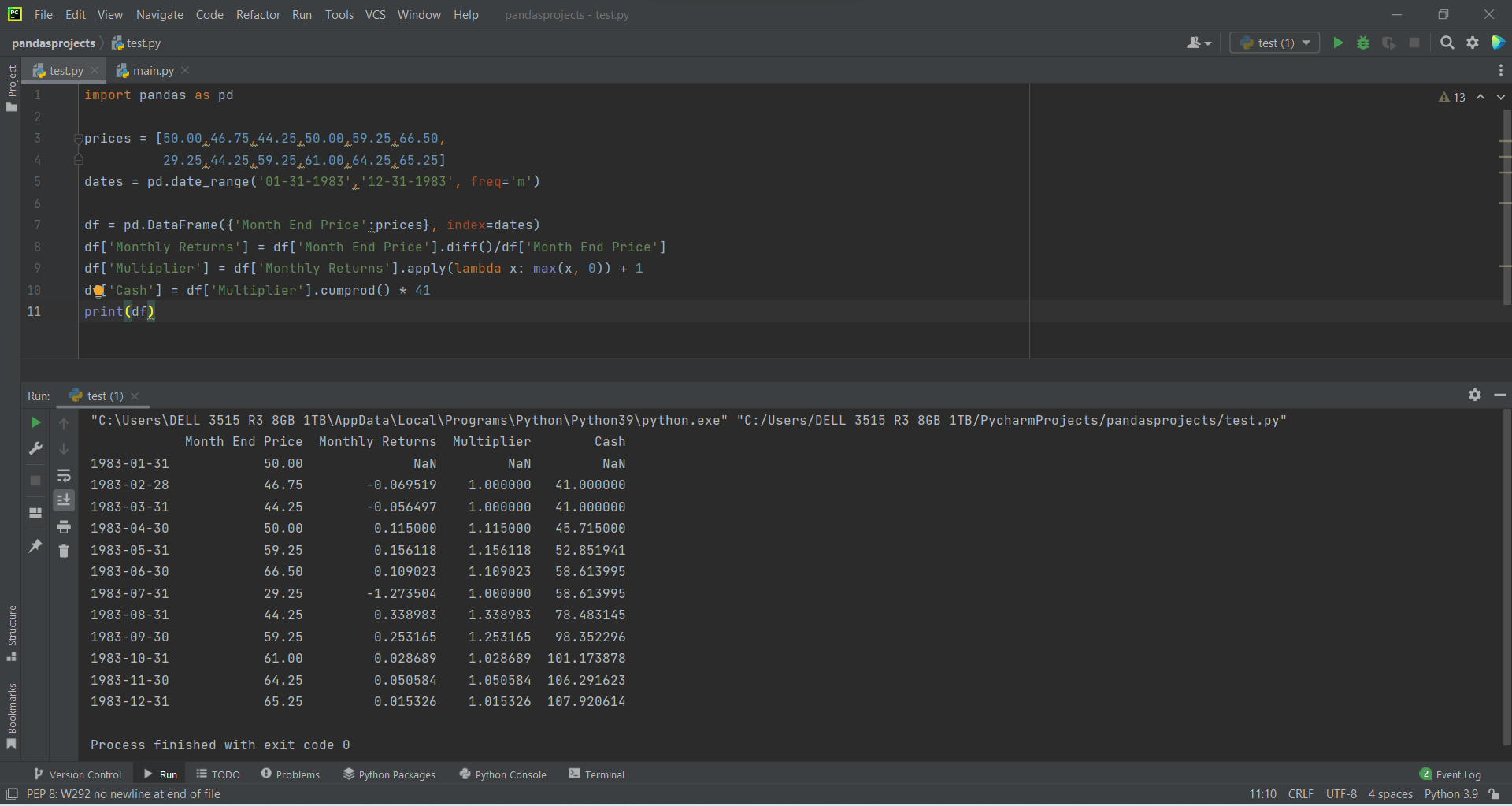# Accumulates returns-forecasting Prices using Pandasby Abdul Rawoof A RUpdated: Feb 20, 2023Solution Kit

To accumulate returns forecasting prices using Pandas, we need one important function called cumprod()

The cumprod() method goes through the values in the DataFrame, from the top, row by row, multiplying the values with the value from the previous row, and ending up with a DataaFrame where the last row contains the product of all values for each column in Python. It is used to get the cumulative product over a data frame or series axis, and it returns a data frame or series of the same size containing the cumulative product in Pandas. For example, we will see how to calculate cumulative returns from annual returns. For that, divide the final value by the initial value, then raise that number by one divided by n (1/n), where 'n' is the number of years you held the investments. Finally, subtract one and multiply by 100. The main purpose of the accumulation function is to measure the signed area in the region between and -axis and play the role of a "place-holder" that allows us to evaluate. The specific number that we are using to bind the region that will determine the area between and the -axis.

Types of price accumulations:

1. Self accumulation.
2. Propagating accumulation.
3. Circulating accumulation.
4. Parallel accumulation.

Here is an example of how to accumulate return-forecasting price using Pandas:Fig : Preview of the output that you will get on running this code from your IDE.

### Code

In this solution we're using Pandas library.

### Instructions

Follow the steps carefully to get the output easily.

2. Copy the snippet using the 'copy' and paste it in your IDE.
3. Add required dependencies and import them in Python file.
4. Run the file to generate the output.

I hope you found this useful. I have added the link to dependent libraries, version information in the following sections.

I found this code snippet by searching for 'how to accumulate returns forecasting using pandas' in kandi. You can try any such use case!

### Environment Tested

I tested this solution in the following versions. Be mindful of changes when working with other versions.

1. The solution is created in PyCharm 2021.3.
2. The solution is tested on Python 3.9.7.
3. Pandas version-v1.5.2.

Using this solution, we are able to accumulate returns-forecasting prices using pandas with simple steps. This process also facilities an easy way to use, hassle-free method to create a hands-on working version of code which would help us to accumulate returns-forecasting prices using pandas.

### Dependent Library

pandasby pandas-dev

Python37415Version:v2.0.0rc1Flexible and powerful data analysis / manipulation library for Python, providing labeled data structures similar to R data.frame objects, statistical functions, and much more

Support
Quality
Security
Reuse

pandasby pandas-dev

Python37415Version:v2.0.0rc1License: Permissive (BSD-3-Clause)

Flexible and powerful data analysis / manipulation library for Python, providing labeled data structures similar to R data.frame objects, statistical functions, and much more
Support
Quality
Security